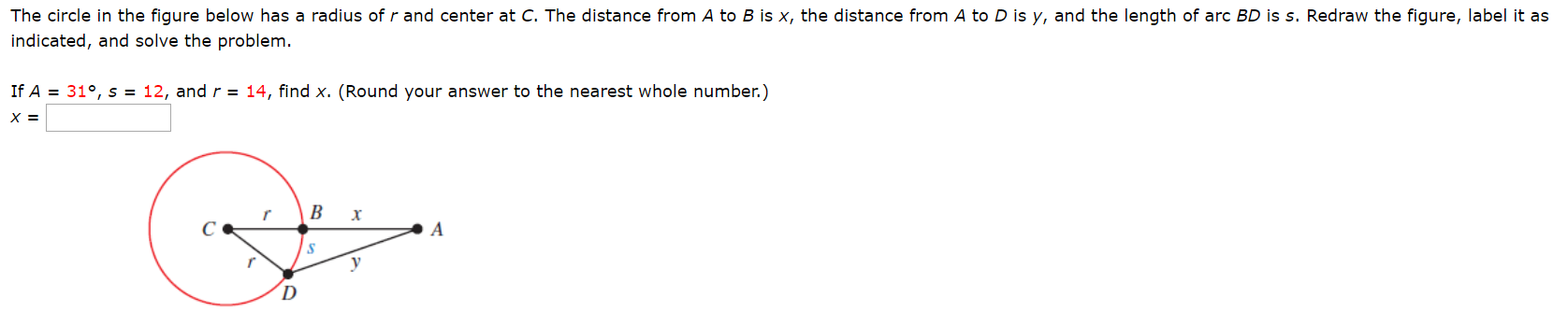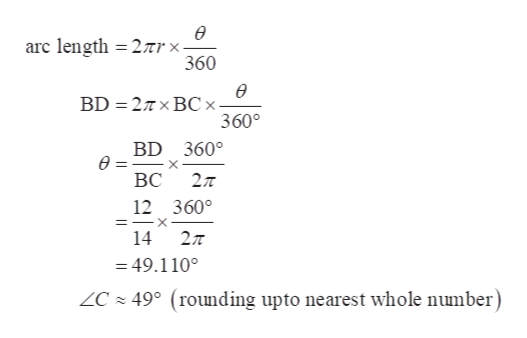# The circle in the figure below has a radius of r and center at C. The distance from A to B is x, the distance from A to D is y, and the length of arc BD is s. Redraw the figure, label it asindicated, and solve the problem.If A31°, s = 12, and r = 14, find x. (Round your answer to the nearest whole number.)х 3ВхAу

Question
26 views

Photo attachedhelp_outlineImage TranscriptioncloseThe circle in the figure below has a radius of r and center at C. The distance from A to B is x, the distance from A to D is y, and the length of arc BD is s. Redraw the figure, label it as indicated, and solve the problem. If A 31°, s = 12, and r = 14, find x. (Round your answer to the nearest whole number.) х 3 В х A у fullscreen
check_circle

star
star
star
star
star
1 Rating
Step 1

First of all, redraw the figure and put the given values.

AB = x

BC = CD = 14

A = 31°

Arc BD = 12

Step 2

Now using the arc length BD to find C.help_outlineImage Transcriptioncloseө arc length 2Trx- 360 ө 2T x BCx- 3600 BD BD 360° ө ВС 27T 12 360° 14 = 49.110° ZC49° (rounding upto nearest whole number) fullscreen
Step 3

Now find D using the sum of angles of a triangle law in ...

### Want to see the full answer?

See Solution

#### Want to see this answer and more?

Solutions are written by subject experts who are available 24/7. Questions are typically answered within 1 hour.*

See Solution
*Response times may vary by subject and question.
Tagged in

### Other